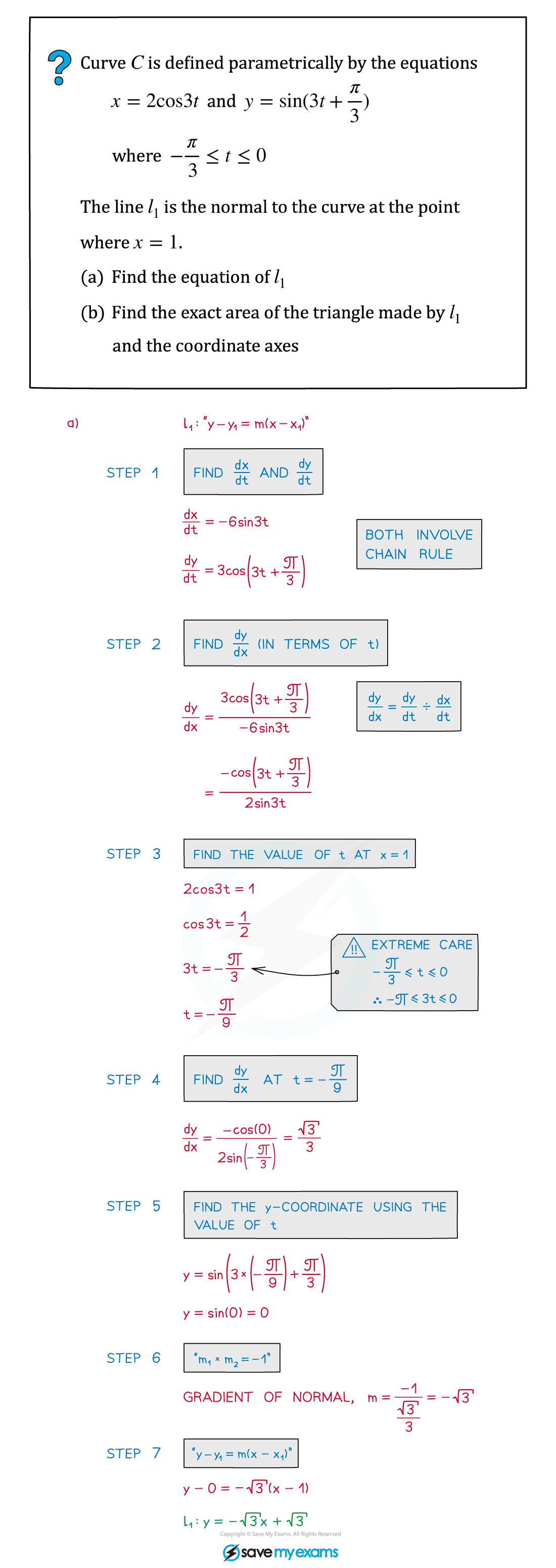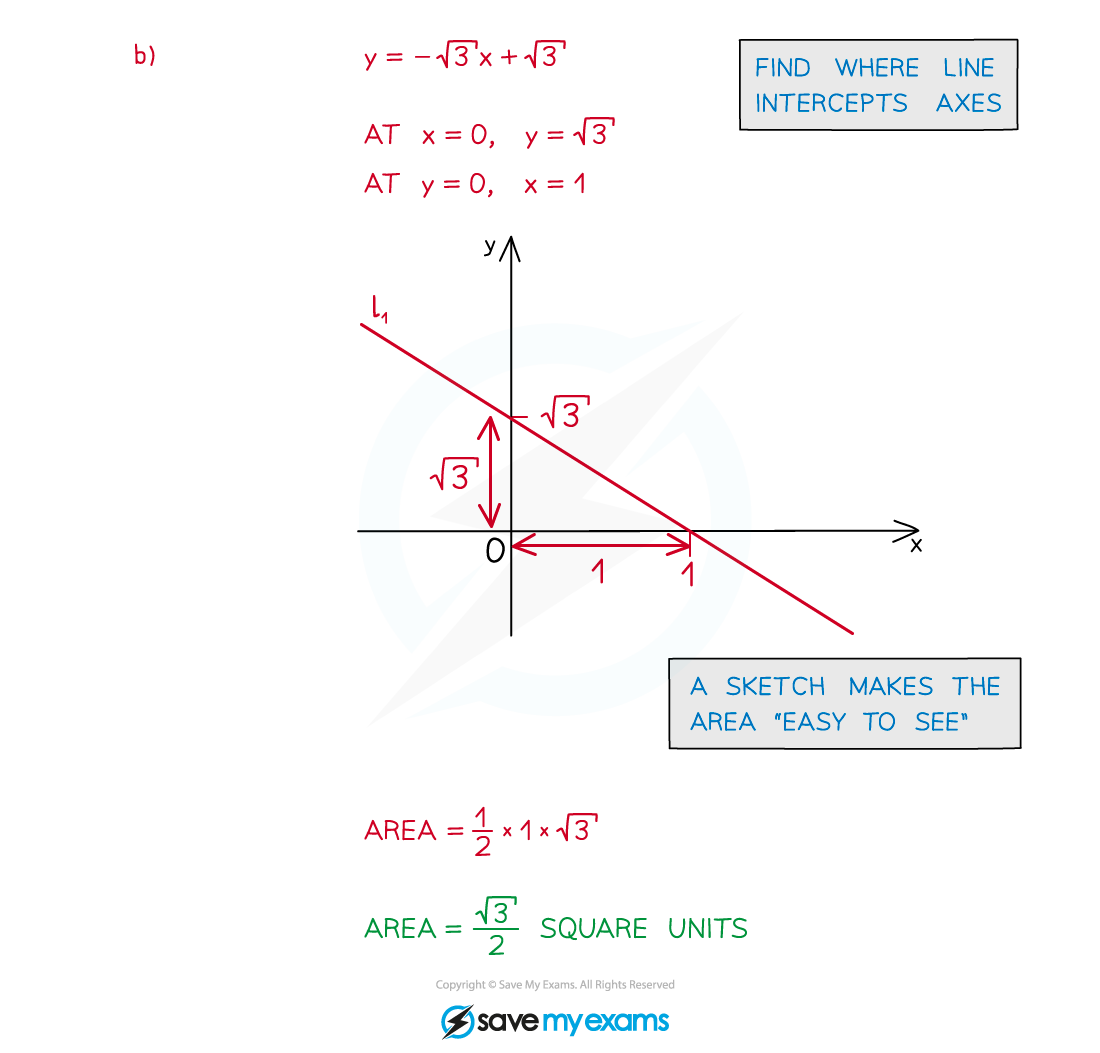# CIE A Level Maths: Pure 3复习笔记4.3.4 Parametric Differentiation

### Parametric Differentiation

#### How do I find dy/dx from parametric equations?

• Ensure you are familiar with Parametric Equations – Basics first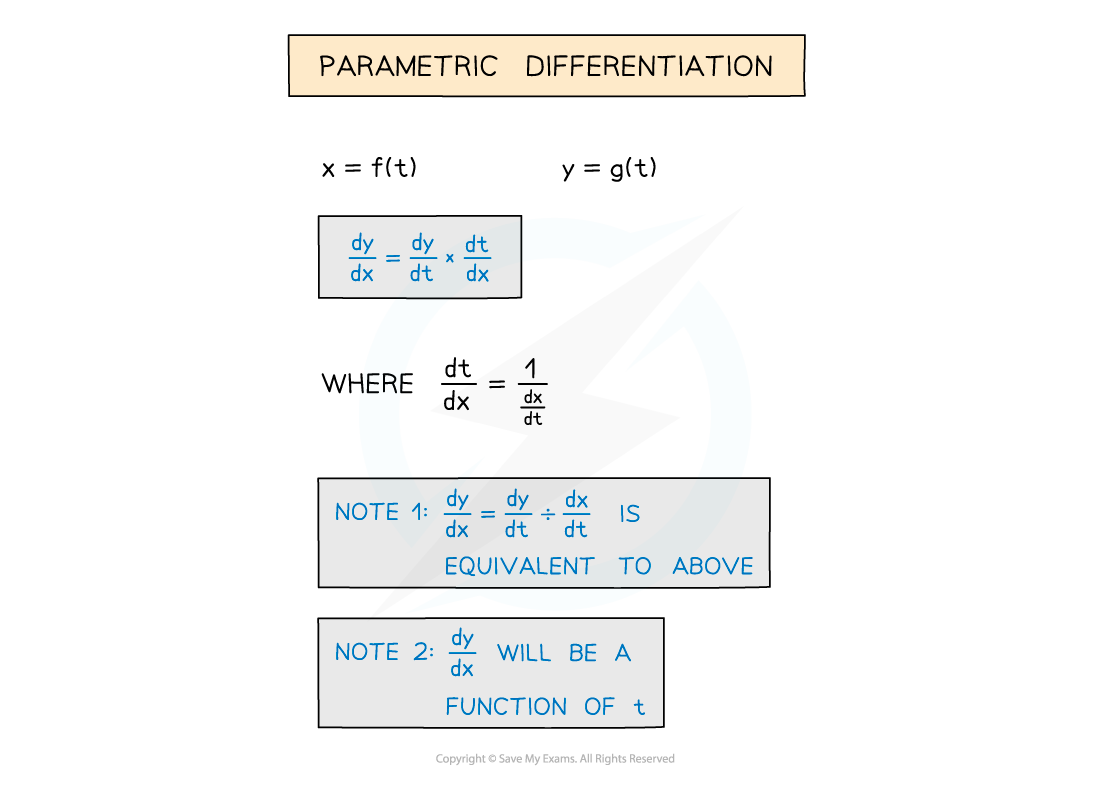• This method uses the chain rule and the reciprocal property of derivatives
•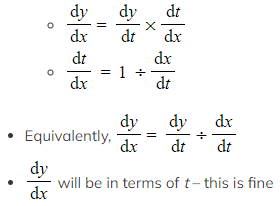Questions usually involve finding gradients, tangents and normals
• The chain rule is always needed when there are three variables or more – see Connected Rates of Change

#### How do I find gradients, tangents and normals from parametric equations?

• To find a gradient …
• STEP 1: Find dx/dt and dy/dt
• STEP 2: Find dy/dx in terms of t

Using either dy/dx = dy/dt ÷ dx/dt

or dy/dx = dy/dt × dt/dx where dt/dx = 1 ÷ dx/dt

• STEP 3: Find the value of t at the required point
• STEP 4: Substitute this value of t into dy/dx to find the gradient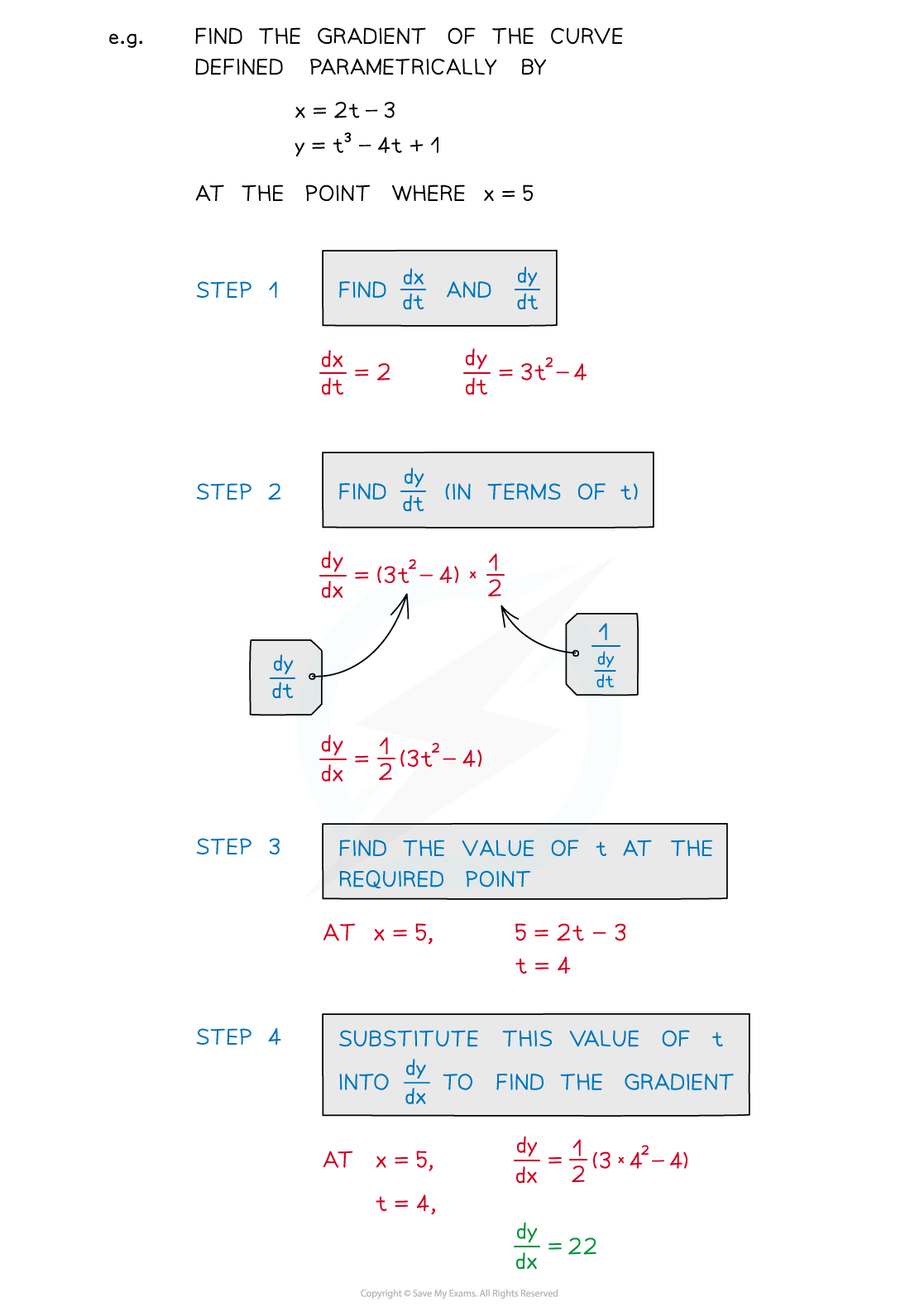• to then go on to find the equation of a tangent …
• STEP 5: Find the y coordinate
• STEP 6: Use the gradient and point to find the equation of the tangent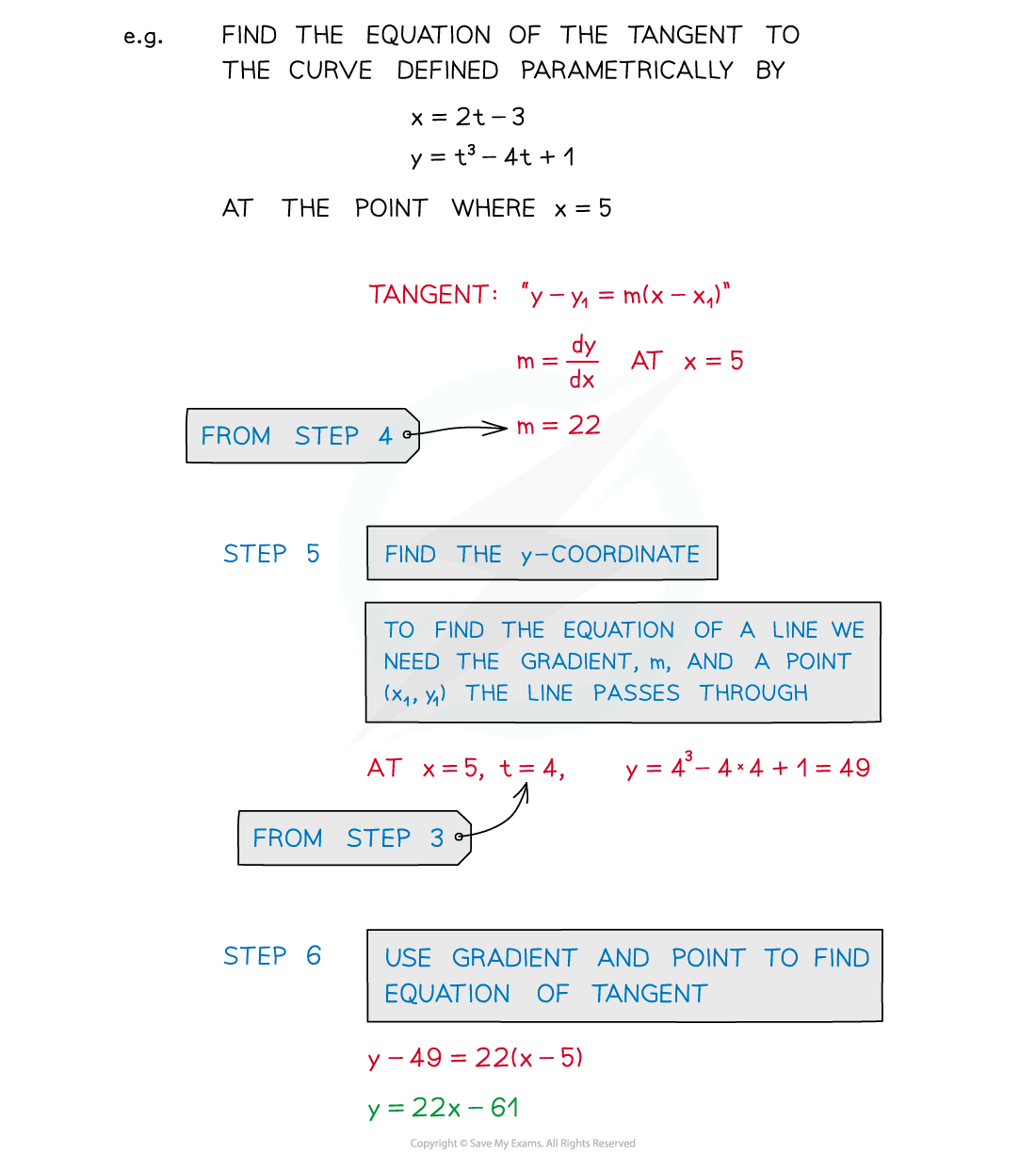• To find a normal...
• STEP 7: Use perpendicular lines property to find the gradient of the normal m1 × m2 = -1
• STEP 8: Use gradient and point to find the equation of the normal y - y1 = m(x - x1)

#### What else may I be asked to do?

• Questions may require use of tangents and normals as per the coordiante geometry sections
• Find points of intersection between a tangent/normal and x/y axes
• Find areas of basic shapes enclosed by axes and/or tangents/normal
• Find stationary points (dy/dx = 0)• You may also be asked about horizontal and vertical tangents
• At horizontal (parallel to the x-axis) tangents, dy/dt = 0
• At vertical (parallel to y-axis) tangents, dx/dt = 0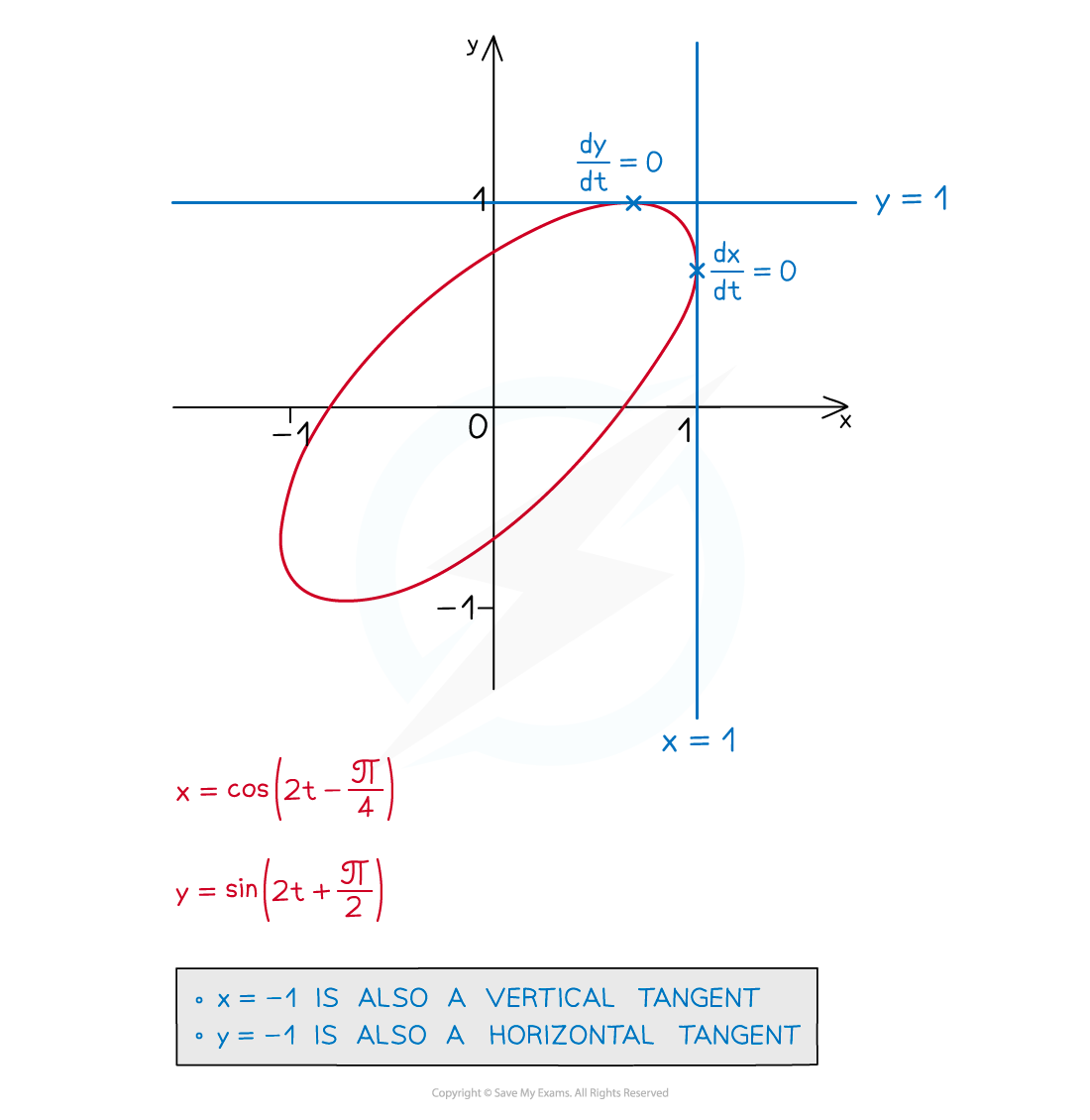#### Just for fun …

• Try plotting the graph from the question below using graphing software
• Plenty of free online tools do this – for example Desmos and Geogebra
• Try changing the domain of t to -π/3 ≤ t ≤ π/3

#### Worked Example